# Zhiwei

Zhiwei wants to divide 19 liters of water equally into 6 pots. Find the volume of water in each pot?

x =  3.1667 l

### Step-by-step explanation: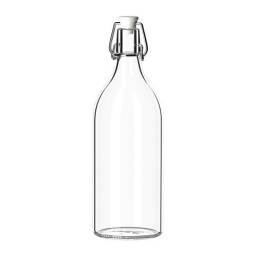Did you find an error or inaccuracy? Feel free to write us. Thank you!Tips to related online calculators
Tip: Our volume units converter will help you with the conversion of volume units.

## Related math problems and questions:

• I have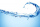I have 1/5 of an ounce of water left. I separate the water equally into two cups. How many ounces of water are in each cup?
• Water tanksA different amount of water is poured into each of the three identical vessels. In the first vessel, water fills 30% of its volume and in the second vessel 40% of its volume. The third container contains 19 liters of water. If we distributed the water fro
• The potThe pot is in 1/3 filled with water. Bottom of the pot has an area of ​​329 cm2. How many centimeters rises water level in the pot after add 1.2 liters of water?
• RegroupingSubtract mixed number with regrouping: 11 17/20- 6 19/20
• Barbara 2Barbara get 6 pizzas to divide equally among 4 people. How much of a pizza can each person have?
• Covid-19 Pandemic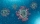Ellice and Zia will write a research on Covid-19 Pandemic. Ellice spends 2 2/4 hours and Zia spends 3 ½ hours. How long does it take them to conduct the research for their projects?
• Bath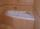In the bath is 30 liters of hot water. Then added 36 liters of cold water at temperature of 19 °C decreased temperature of water to 41 °C. What was the initial temperature of the hot water?
• FractionsSort fractions z1 = (6)/(11); z2 = (10)/(21); z3 = (19)/(22) by its size. Result write as three serial numbers 1,2,3.
• Dividing moneyJane and Silvia are to divide 1200 euros in a ratio of 19:11. How many euros does Jane have?
• Like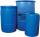When is in 14 barrels 140 liters of water, how many liters barrels of 8 liters I need to get all the water from the larger barrels?
• PlumsIn the bowl are plums. How many would be there if we can divide it equally among 8, 10 and 11 children?
• Water 31Richard takes 3 1/6 liters of water before noon and 2 3/5 liters of water afternoon. How many liters of water does Richard consume a day?
• Pie division5/8 of a pie divide 6 pieces. Each friend got 1/6.  What fraction  of the whole pie does each person receive?
• Peroxide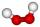How much distilled water (in liters) must pharmacists pour into 300 ml of 23.6% solution of hydrogen peroxide to get 2.7% solution to gargle?
• Paper collecting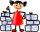At the paper collecting contest gathered Franta 2/9 ton, Karel 1/4 ton, and Patrick 19/36 tons of paper. Who has gathered the most and the least?
• Water tank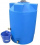The block-shaped tank is 2.5 m long, 100 cm wide and 12 dm high. In how many minutes will it be filled with water to two thirds if 40 liters of water flow into the tank per minute?
• Empty barrel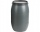In the backyard is an empty barrel with a volume of 2 hl. How many liters are missing at the edge, when 53 liters flowed into it for 1 day and 68 liters of water for 2 days?